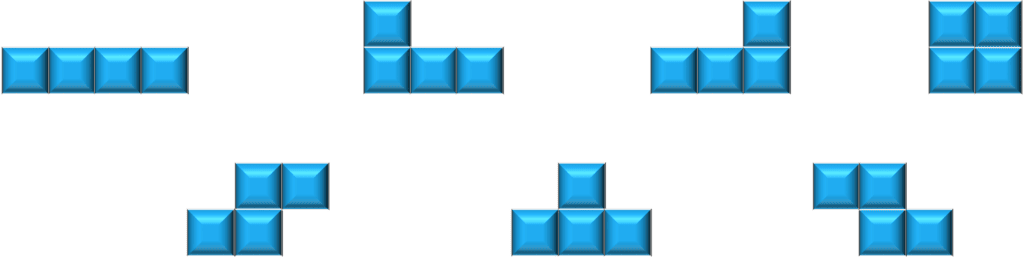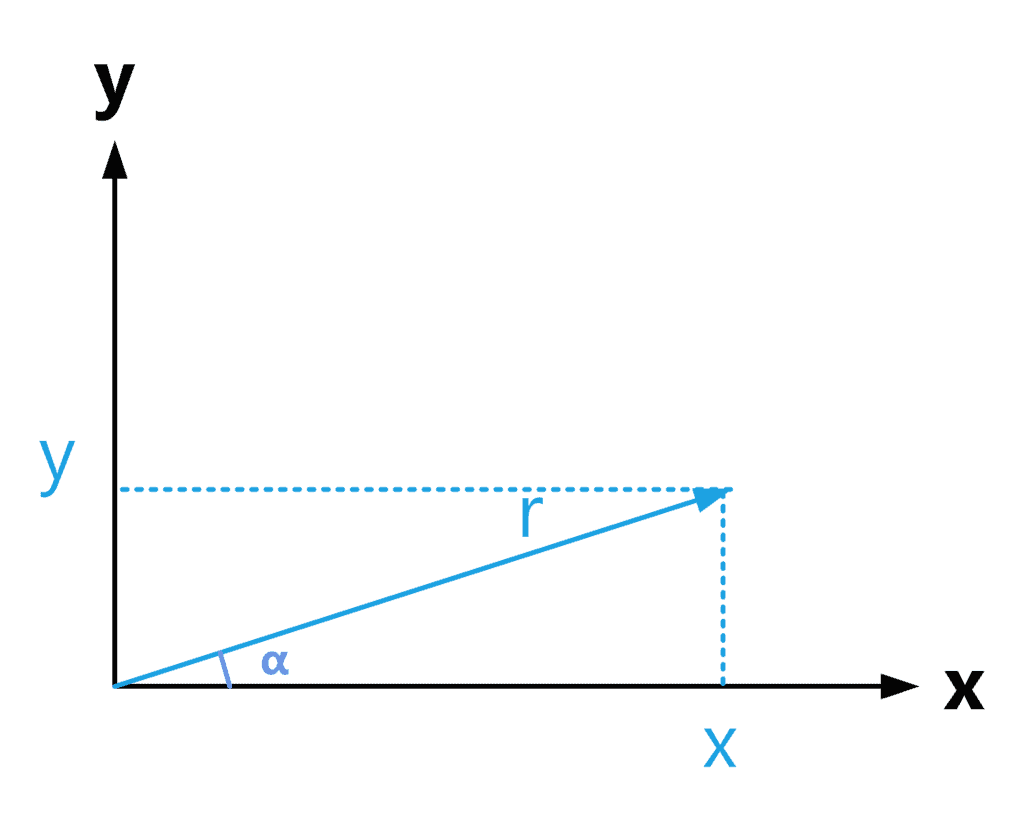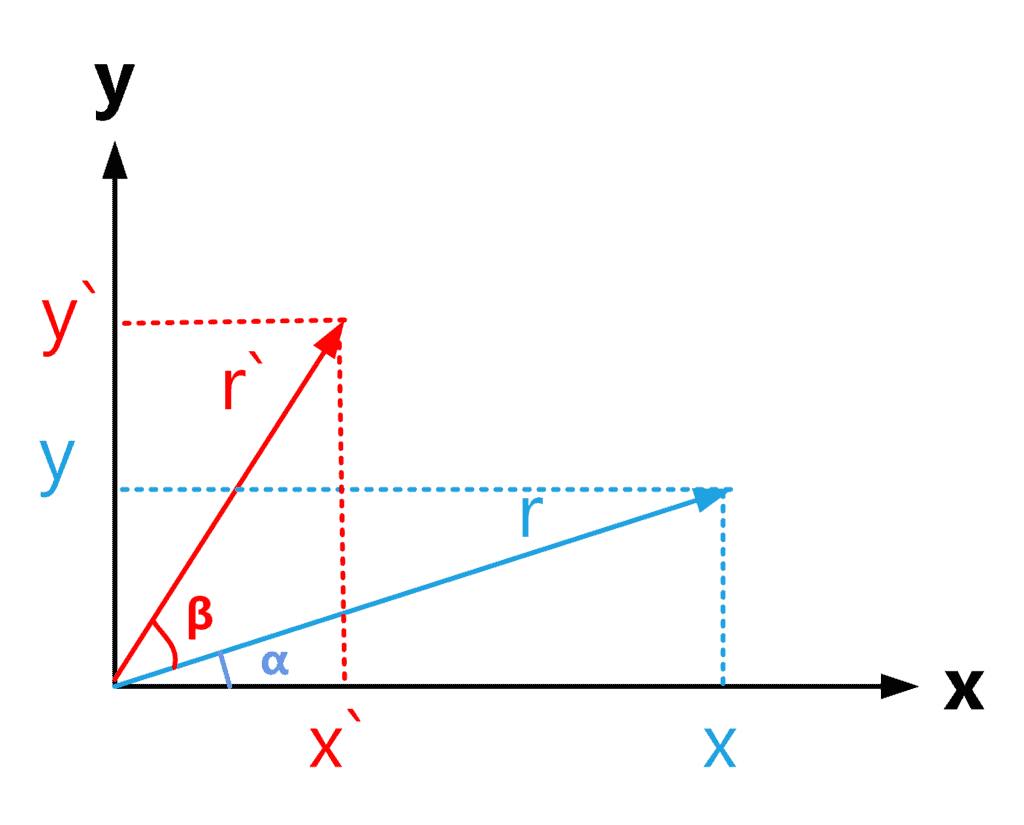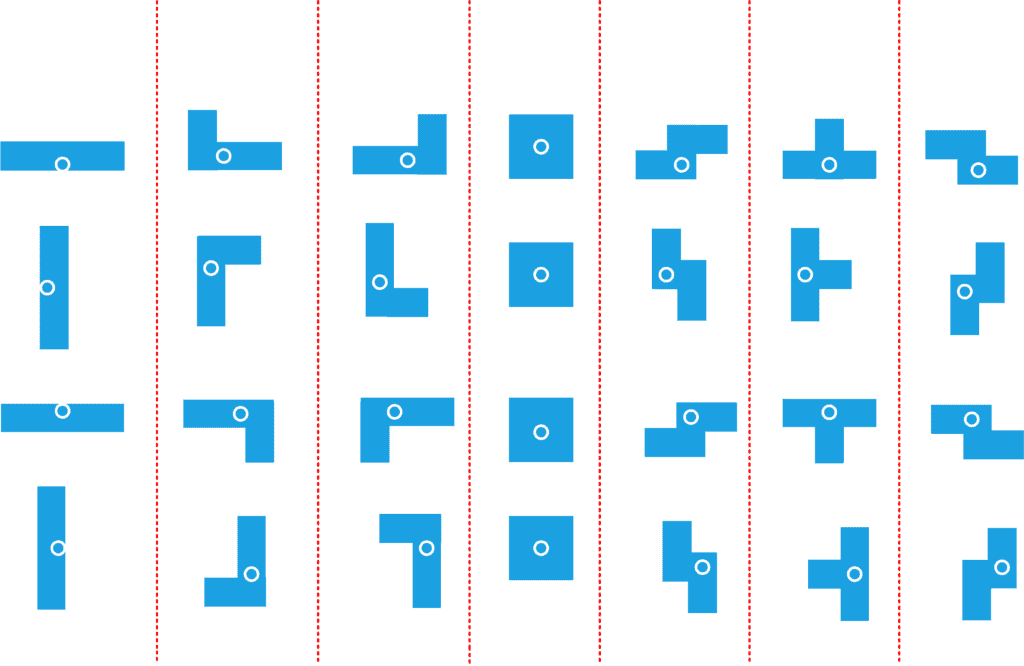## 1. Overview

In this tutorial, we’ll discuss the algorithm behind rotating Tetris pieces. We’ll start by discussing the equation for rotating a shape in general. Then, we’ll define a data structure that can be used to manipulate the Tetris pieces’ rotations.

Finally, we’ll present the algorithm to rotate a Tetris piece and finish with a quick conclusion.

## 2. Rotating a Shape

First, let’s define the problem from a logical and mathematical point of view. Then, we can extract the general equations to rotate a point in the cartesian coordinate system.

### 2.1. Defining the Problem

The Tetris game consists of the following 7 main shapes:Each shape can be rotated and then put on top of others to form straight lines. The allowed rotations for each piece are 90, 180, 270, and 360 degrees, which is the original orientation of the shape. This tutorial discusses the general algorithm to rotate any of the mentioned pieces.

The algorithm should rotate a given Tetris piece by 90 degrees. Calling the algorithm multiple times will generate all the possible rotations for the given piece. As a start, let’s discuss the general rotation equations and then see how to apply them in the intended algorithm.

### 2.2. Mathematical Representation

Since each Tetris piece is a polygon, rotating each of the polygon’s corners alone should give us the resulting rotated piece. Therefore, in this section, we’ll discuss the equations to rotate a point around the origin of the cartesian coordinate axis.

Let’s take a look at the following figure:In the coordinates above, we have pointforming an anglewith the x-axis. For simplicity, we’ll consider the point as a vector with magnitude. Using this representation, we can define equations forandas follows:### 2.3. Rotating a Point

After defining the equations to represent a point in the coordinate axis, let’s rotate the pointto new coordinates. The rotation is shown in the following shape:The original point was rotated bydegrees to the new coordinateshaving a new magnitude of. We can see that the new point forms an anglewith the x-axis. Likewise, we can define equations foras follows:### 2.4. Rotation Equations

To find the rotation equations, we need to findandas functions to the original coordinatesandTo do this, we’ll use the following known equations ofandfor the sum of two angles:Additionally, rotating a vector doesn’t change its magnitude. Therefore, the following equation applies:Now, we can use the above equations to rewrite our formula forand:We can simplify them using the equations we defined in section 2.2 to the following ones:Now, we can use these equations to create an algorithm that can rotate a Tetris piece. Let’s start by defining the data structure to store the pieces.

## 3. Structure Definition

We need to define the structure that will store the Tetris pieces. To do that, we can define each shape as a set of points which are the corners of the shape. In addition, the structure must support rotating the Tetris pieces.

It’s worth noting that Tetris pieces are rotated around their origin. Therefore, we need to define the center point for each shape as well. Take a look at the following figure that shows the different rotations of each shape along with its origin:Therefore, for each shape, we’ll have an arraycontaining the shape’s corner points and a variablecontaining the shape’s origin. Now that we defined the structure, we can move into implementing the rotation algorithm.

## 4. Algorithm

To implement a rotation algorithm, we only need one function called, which rotates the given shape by the given angle. Note that the piece is not initially located at the coordinated axis’s center. Therefore, the algorithm must shift the shape to the center of the coordinate axis, perform the rotations, and then shift the point back.

Let’s take a look at the algorithm:The function takes the array of points, the origin of the shape, and the rotation angleas input. We start by defining, which will hold the resulting rotated points. Note that the origin stays the same after the rotation, so we don’t need to return it.

Next, we iterate over all the points of the shape. We need to shift each point as if the shape’s origin is moved to the centre of the coordinate axis. If the origin is moved to the centre, then it shifts to point. At this time, the point will also shift with the same amount. Therefore, we shift the point by. As a result, we defineand, which are the shifted coordinates.

Then, we perform the rotation by definingandwhich are the coordinates for the rotated point, by applying the equations from section 2. After that, we shift the new pointsandback away from the centre of the coordinate axis. Finally, we add the new points to the list of rotated points we created.

In the end, we return the resultingas the new shape corder points after performing the rotation.

## 5. Conclusion

In this article, we discussed the algorithm for rotating Tetris pieces. We started by discussing the rotation problem in general and then moved to define the structure that stores the Tetris pieces and the algorithm for rotating them.